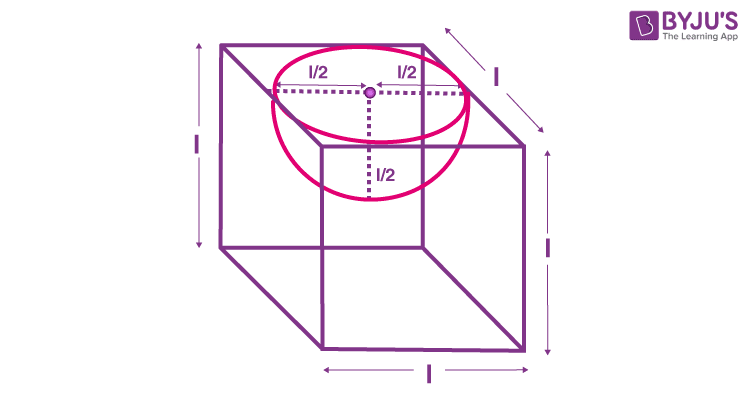# A hemispherical depression is cut out from one face of a cubical wooden block such that the diameter l of the hemisphere is equal to the edge of the cube. Determine the surface area of the remaining solid.

Diameter of the hemisphere is equal to edge of the cube

Thus, diameter = side of cube = l

Surface area of solid = Area of cube + Curved Surface area of hemisphere – Base area of hemisphere

Area of cube = 6l2

Curved syrface area of hemisphere = 2πr2r = l/2

Therefore,

CSA (Hemisphere) = 2π(l/2)2 = π/2 l2

Now,

Base area of hemisphere = πr2 = π(l/2)2 = π/4 l2

Hence, Surface area of solid is;

SA = Area of cube + Curved Surface area of hemisphere – Base area of hemisphere

SA = 6l2 + π/2 l2 – π/4 l2

SA = l2/4 (π+24) sq.unit.